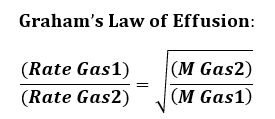# Problem: Two identical balloons are filled to the same volume, one with air and one with helium. The next day, the volume of the air-filled balloon has decreased by 5.1 % .By what percent has the volume of the helium-filled balloon decreased? (Assume that the air is four-fifths nitrogen and one-fifth oxygen, and that the temperature did not change.)

🤓 Based on our data, we think this question is relevant for Professor Young's class at UCSD.

###### FREE Expert Solution

Effusion involves a gas escaping a container through an opening. And based on Graham’s Law of Effusion, we can compare the rate of the two gases.Calculate molar mass of air:###### Problem Details

Two identical balloons are filled to the same volume, one with air and one with helium. The next day, the volume of the air-filled balloon has decreased by 5.1 % .

By what percent has the volume of the helium-filled balloon decreased? (Assume that the air is four-fifths nitrogen and one-fifth oxygen, and that the temperature did not change.)